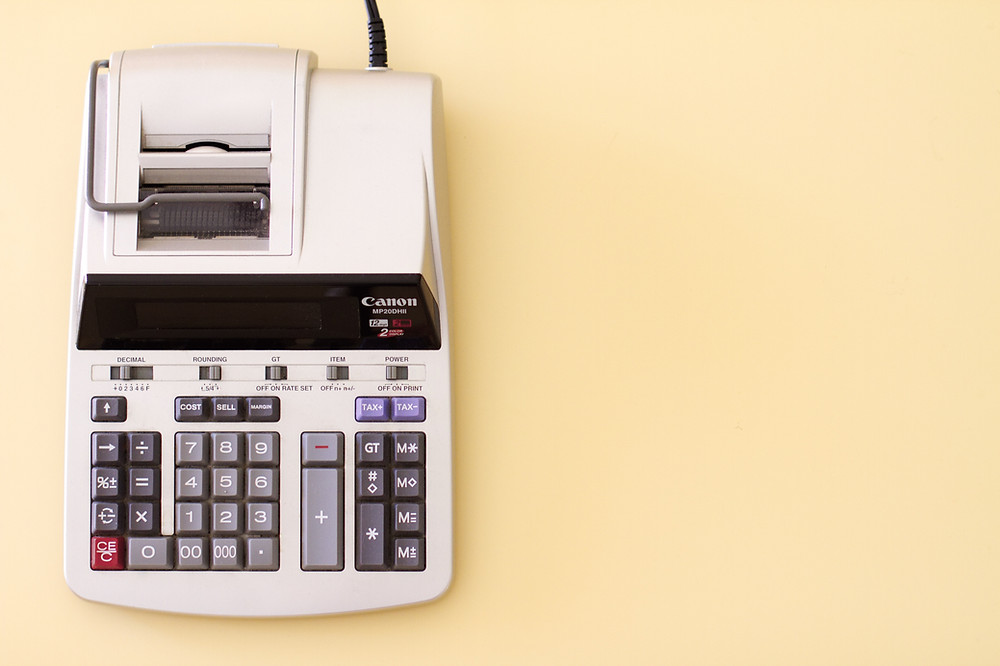Search

# How to Calculate Depreciation as per Schedule II of Companies Act

An overview of Depreciation under Schedule II of Companies Act, 2013

The value of fixed assets such as cars, machinery, buildings etc. are reduced over their economic lifetime due to wear and tear or passage of time. The process of reduction in the value over the period of time is called Depreciation. There are majorly two accounting policies or method for depreciation (i.e. Straight-line method or Written down value method) given in the accounting standards issued by ICAI. However, for the purpose of useful life we have to look into the schedule II of the companies Act, 2013 and on the basis of the useful life given thereunder we need to calculate the applicable rate on the assets.1. Methods of computing depreciation

2. Provisions specified under Schedule II of Companies Act, 2013

3. Useful life & residual value of assets

4. Non applicability of depreciation under Companies Act

5. Component Depreciation Accounting

6. Double and Triple shifts depreciation

7. Benefits of charging depreciation

8. Illustration for charging depreciation

### 1. Methods of computing depreciation

There are two methods of calculating depreciation

Straight line method -

Where the amount of depreciation over the years would remain same through out the life of the assets

Computation under Straight line method

Calculation of depreciation under the SLM method is like Simple Interest calculation where the depreciation is the same till the end of the useful life of the asset. Formula for calculation is

Depreciation = Cost of the asset- Residual Value

Life of the asset

Written down value method-

Under this method depreciation amount goes on decreasing as it is calculated on carrying value of the asset

Computation under Written Down Value method

Similar to compound interest calculation, the amount of depreciation under the WDV method keeps decreasing. In this method book value of assets is relevant, not the original cost. Formula for calculation is

Depreciation = Carrying value of asset×Rate of depreciation

Rate of depreciation = {1-(s/c)^(1/n)}*100

S = Scrap value at the end of the useful life of assets

C = Cost of assets

n = Useful life of assets given under schedule II of companies Act (Will discuss below)

### 2. Provisions specified under Schedule II of Companies Act, 2013

● Earlier before companies act, 2013 rate of depreciation was prescribed.

● However, under the new companies act the rates are not prescribed and calculation is made by considering useful life of the assets and its residual value

● A company cannot change the method of computing depreciation from SLM to WDV or vice versa.

● Charging depreciation is mandatory under Companies Act, 2013

● If the cost if an asset is up to Rs 5000, depreciation should be charged @100%

### 3. Useful life & residual value of assets

Part C of Schedule 2 states the useful life for different assets. The useful life shall not be more than that prescribed under Part C of Schedule 2. In case if there is any changes, then it should be justified by disclosing it in the financial statement with prior approval from the management or technical validation.

It is the scrap value of the asset at the end of its useful life. Under Schedule II of Companies Act, 2013 it is stated that the residual value should not be more than 5% of original cost of the asset. If the residual value is more than 5% then the approval of the management is required or technical validation should be there.

The following assets life has been given in the schedule II of companies Act, 2013

### 4. Non applicability of depreciation under Companies Act

Provisions of depreciation under Companies Act 2013 is only applicable on tangible assets. For intangible assets and amortisation of assets AS 26 or Ind AS 38 is to be followed

### 5. Component Accounting

Useful life stated in Part C of Schedule II is for the whole of the asset. But in cases where a part of an asset is significant it should be separated from the actual asset. It is also applicable when part of an asset is sold or an additional unit is purchased. Depreciation for such parts should be computed separately according to date of purchase or sale of asset. . In case of component accounting date of purchase of the asset should be considered while computing depreciation

### 6. Double and Triple shifts depreciation

● The Useful life mentioned in Companies Act, 2013 are only relevant to single shift assets

● For double shifts, 50% additional depreciation is to be charged

● For tripe shifts, 100% additional depreciation should be charged

### 7. Benefits of charging depreciation

1. Depreciation generates tax savings

2. True value of asset can be calculated

3. Charging deprivation helps in recovery of purchase cost of assets

### 8. Illustration for charging depreciation

A machine costing Rs. 50,000 with a useful life of 5 years and scrap value Rs. 2000 is purchased. If the rate of depreciation is 10% (i.e. Computed on the basis of formula discussed above) compute depreciation under SLM and WDV method

Under straight line method,

Depreciation= 50,000-2,000

5

= Rs 9,600 per year for whole life of the assets

Under Written Down Value method

For first year

Depreciation= 50,000×10%

= Rs 5,000

For second year

Depreciation= (50,000-5,000)×10%

= Rs 4,500

For third year

Depreciation= (45,000-4,500)×10%

= Rs 4,050

and so on keep reducing

So under the Straight Line Method the same amount of depreciation is charged for all years. Under WDV, the amount of depreciation keeps diminishing over the useful life of assets. A company can choose a method of computation based on its useful life and repair cost to be incurred.